# Excel DATEDIF to calculate date difference in days, weeks, months or years

In this tutorial, you will find a simple explanation of the Excel DATEDIF function and a few formula examples that demonstrate how to compare dates and calculate the difference in days, weeks, months or years.

Over the past few weeks, we investigated nearly every aspect of working with dates and times in Excel. If you have been following our blog series, you already know how to insert and format dates in your worksheets, how to calculate weekdays, weeks, months and years as well as add and subtract dates.

In this tutorial, we will focus on calculating date difference in Excel and you will learn different ways to count the number of days, weeks, months and years between two dates.

## Excel DATEDIF function - get date difference

As its name suggests, the DATEDIF function is purposed for calculating the difference between two dates.

DATEDIF is one of very few undocumented functions in Excel, and because it is "hidden" you won't find it on the Formula tab, nor will you get any hint on which arguments to enter when you start typing the function's name in the formula bar. That is why it's important to know the complete syntax of Excel DATEDIF to be able to use it in your formulas.

#### Excel DATEDIF function - syntax

The syntax of the Excel DATEDIF function is as follows:

DATEDIF(start_date, end_date, unit)

All three arguments are required:

Start_date - the initial date of the period you want to calculate.

End_date - the ending date of the period.

Unit - the time unit to use when calculating the difference between two dates. By supplying different units, you can get the DATEDIF function to return the date difference in days, months or years. Overall, 6 units are available, which are described in the following table.

 Unit Meaning Explanation Y Years Number of complete years between the start and end dates. M Months Number of complete months between the dates. D Days Number of days between the start date and end date. MD Days excluding years and months The date difference in days, ignoring months and years. YD Days excluding years The date difference in days, ignoring years. YM Months excluding days and years The date difference in months, ignoring days and years.

## Excel DATEDIF formula

To get the difference between two dates in Excel, your main job is to supply the start and end dates to the DATEDIF function. This can be done in various ways, provided that Excel can understand and correctly interpret the supplied dates.

Cell references

The easiest way to make a DATEDIF formula in Excel is to input two valid dates in separate cells and refer to those cells. For example, the following formula counts the number of days between the dates in cells A1 and B1:

`=DATEDIF(A1, B1, "d")`

Text strings

Excel understands dates in many text formats such as "1-Jan-2023", "1/1/2023", "January 1, 2023", etc. The dates as text strings enclosed in quotation marks can be typed directly in a formula's arguments. For instance, this is how you can calculates the number of months between the specified dates:

`=DATEDIF("1/1/2023", "12/31/2025", "m")`

Serial numbers

Since Microsoft Excel stores each date as a serial number beginning with January 1, 1900, you use numbers corresponding to the dates. Although supported, this method is not reliable because date numbering varies on different computer systems. In the 1900 date system, you can use the below formula to find the number of years between two dates, 1-Jan-2023 and 31-Dec-2025:

`=DATEDIF(44927, 46022, "y")`

Results of other functions

To find out how many days there are between today and 20 May, 2025, this is the formula to use.

`=DATEDIF(TODAY(), "5/20/2025", "d")`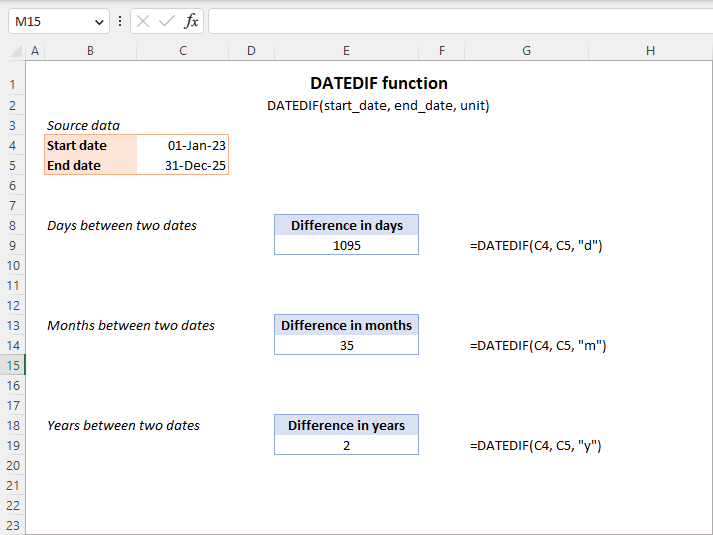Note. In your formulas, the end date must always be greater than the start date, otherwise the Excel DATEDIF function returns the #NUM! error.

Hopefully, the above information has been helpful to understand the basics. And now, let's see how you can use the Excel DATEDIF function to compare dates in your worksheets and return the difference.

## How to get the number of days between two dates in Excel

If you observed DATEDIF's arguments carefully, you've noticed that there exist 3 different units for counting days between the dates. Which one to use depends on exactly what your needs are.

#### Example 1. Excel DATEDIF formula to calculate date difference in days

Supposing you have the start date in cell A2 and the end date in cell B2 and you want Excel to return the date difference in days. A simple DATEDIF formula works just fine:

`=DATEDIF(A2, B2, "d")`

Provided that a value in the start_date argument is less than in end_date. In case the start date is greater than the end date, the Excel DATEDIF function returns the #NUM error, as in row 5: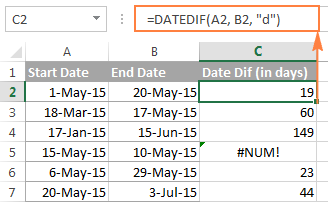If you are looking for a formula that can return the date difference in days as either a positive or negative number, simply subtract one date directly from the other:

`=B2-A2`

Please see How to subtract dates in Excel for full details and more formula examples.

#### Example 2. Count days in Excel ignoring years

Supposing you have two lists of dates that belong to different years and you wish to calculate the number of days between the dates as if they were of the same year. To do this, use a DATEDIF formula with "YD" unit:

`=DATEDIF(A2, B2, "yd")`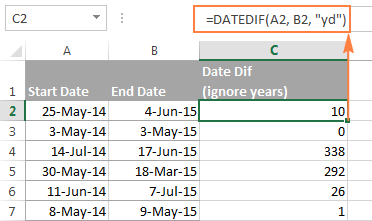If you want the Excel DATEDIF function to ignore not only years but also moths, then use the "md" unit. In this case, your formula will calculate days between two dates as if they were of the same month and the same year:

`=DATEDIF(A2, B2, "md")`

The screenshot below demonstrates the results, and comparing it with the screenshot above can help understand the difference better.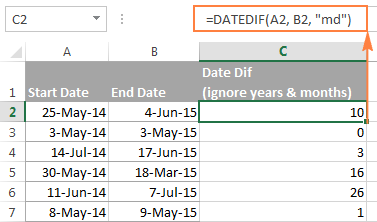Tip. To get the number of working days between two dates, use the NETWORKDAYS or NETWORKDAYS.INTL function.

## How to calculate date difference in weeks

As you probably noticed, the Excel DATEDIF function does not have a special unit to calculate date difference in weeks. However, there is an easy workaround.

To find out how many weeks there are between two dates, you can use the DATEDIF function with "D" unit to return the difference in days, and then divide the result by 7.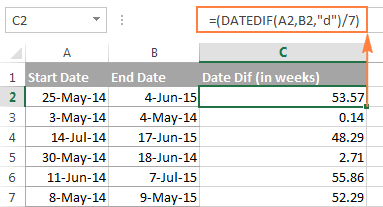To get the number of full weeks between the dates, wrap your DATEDIF formula in the ROUNDDOWN function, which always rounds the number towards zero:

`=ROUNDDOWN((DATEDIF(A2, B2, "d") / 7), 0)`

Where A2 is the start date and B2 is the end date of the period you are calculating.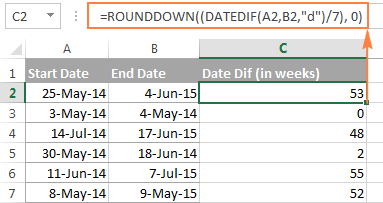## How to calculate the number of months between two dates in Excel

Similarly to counting days, the Excel DATEDIF function can compute the number of months between two dates that you specify. Depending on the unit you supply, the formula will produce different results.

#### Example 1. Calculate complete months between two dates (DATEDIF)

To count the number of whole months between the dates, you use the DATEDIF function with "M" unit. For example, the following formula compares the dates in A2 (start date) and B2 (end date) and returns the difference in months:

`=DATEDIF(A2, B2, "m")`

Note. For the DATEDIF formula to calculate months correctly, the end date should always be greater than the start date; otherwise the formula returns the #NUM error.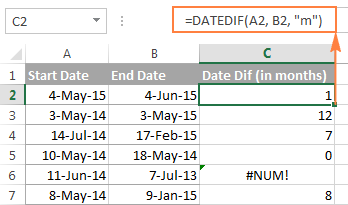To avoid such errors, you may force Excel to always perceive an older date as the start date, and a more recent date as the end date. To do this, add a simple logical test:

`=IF(B2>A2, DATEDIF(A2,B2,"m"), DATEDIF(B2,A2,"m"))`

#### Example 2. Get the number of months between two dates ignoring years (DATEDIF)

To count the number of months between the dates as if they were of the same year, type "YM" in the unit argument:

`=DATEDIF(A2, B2, "ym")`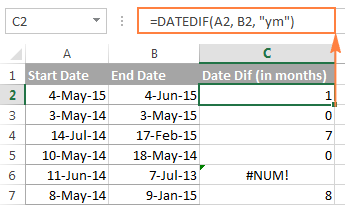As you see, this formula also returns an error in row 6 where end date is less than the start date. If your data set may contain such dates, you will find the solution in the next examples.

#### Example 3. Calculating months between two dates (MONTH function)

An alternative way to calculate the number of months between two dates in Excel is using the MONTH function, or more precisely a combination of MONTH and YEAR functions:

`=(YEAR(B2) - YEAR(A2))*12 + MONTH(B2) - MONTH(A2)`

Of course, this formula is not so transparent as DATEDIF and it does take time to wrap your head around the logic. But unlike the DATEDIF function, it can compare any two dates and return the difference in months as either a positive or negative value: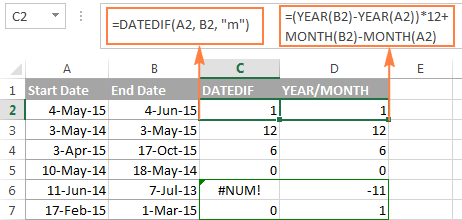Notice that the YEAR/MONTH formula has no problem with calculating months in row 6 where the start date is more recent than the end date, the scenario in which an analogues DATEDIF formula fails.

Note. The results returned by DATEDIF and YEAR/MONTH formulas are not always identical because they operate based on different principles. The Excel DATEDIF function returns the number of complete calendar months between the dates, while the YEAR/MONTH formula operates on months' numbers.

For example, in row 7 in the screenshot above, the DATEDIF formula returns 0 because a complete calendar month between the dates has not elapsed yet, while YEAR/MONTH returns 1 because the dates belong to different months.

#### Example 4. Counting months between 2 dates ignoring years (MONTH function)

In case all of your dates are of the same year, or you want to calculate months between the dates ignoring years, you can the MONTH function to retrieve the month from each date, and then subtract one month from the other:

`=MONTH(B2) - MONTH(A2)`

This formula works similarly to Excel DATEDIF with "YM" unit as demonstrated in the following screenshot: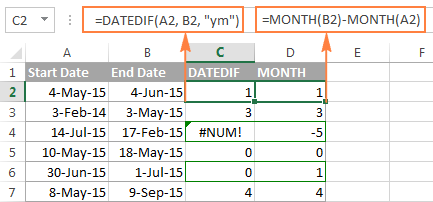However, the results returned by two formulas differ is a couple of rows:

• Row 4: the end date is less than the start date and therefore DATEDIF returns an error while MONTH-MONTH yields a negative value.
• Row 6: the dates are of different months, but the actual date difference is just one day. DATEDIF returns 0 because it calculates whole months between 2 dates. MONTH-MONTH returns 1 because it subtracts the months' numbers from each other ignoring days and years.

## How to calculate years between two dates in Excel

If you followed the previous examples where we calculated months and days between two dates, then you can easily derive a formula to calculate years in Excel. The following examples can help you check if you got the formula right :)

#### Example 1. Calculating complete years between two dates (DATEDIF function)

To find out the number of complete calendar years between two dates, use the old good DATEDIF with the "Y" unit:

`=DATEDIF(A2,B2,"y")`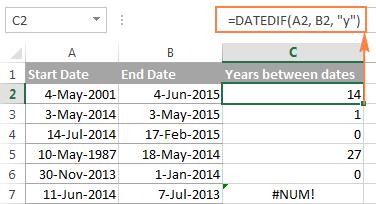Notice that the DATEDIF formula returns 0 in row 6, although the dates are of different years. This is because the number of full calendar years between the start and end dates equals to zero. And I believe you are not surprised to see the #NUM! error in row 7 where the start date is more recent than the end date.

#### Example 2. Calculating years between two dates (YEAR function)

An alternative way to calculate years in Excel is using the YEAR function. Similarly to the MONTH formula, you extract the year from each date, and then subtract the years from each other:

`=YEAR(B2) - YEAR(A2)`

In the following screenshot, you can compare the results returned by the DATEDIF and YEAR functions: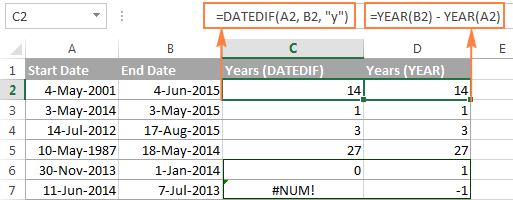In most cases the results are identical, except that:

• The DATEDIF function calculates complete calendar years, while the YEAR formula simply subtracts one year from the other. Row 6 illustrates the difference.
• The DATEDIF formula returns an error if the start date is greater than the end date, while the YEAR function returns a negative value, as in row 7.

## How to get date difference in days, months and years

To count the number of complete years, months and days between two dates in a single formula, you simply concatenate three DATEDIF functions:

`=DATEDIF(A2, B2, "y") &" years, "&DATEDIF(A2, B2, "ym") &" months, " &DATEDIF(A2, B2, "md") &" days"`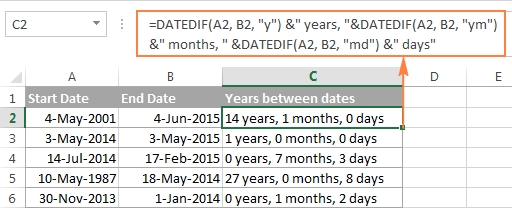If you'd rather not display zero values, you can wrap each DATEDIF in the IF function as follows:

`=IF(DATEDIF(A2,B2,"y")=0, "", DATEDIF(A2,B2,"y") & " years ") & IF(DATEDIF(A2,B2,"ym")=0,"", DATEDIF(A2,B2,"ym") & " months ") & IF(DATEDIF(A2, B2, "md")=0, "", DATEDIF(A2, B2, "md") & " days"`

The formula displays only non-zero elements as demonstrated in the following screenshot: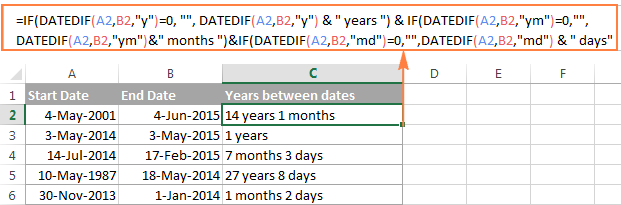For other ways to get date difference in days, see How to calculate days since or until date in Excel.

## DATEDIF formulas to calculate age in Excel

In fact, calculating someone's age based on the date of birth is a special case of calculating date difference in Excel, where the end date is today's date. So, you use a usual DATEDIF formula with "Y" unit that returns the number of years between the dates, and enter the TODAY() function in the end_date argument:

`=DATEDIF(A2, TODAY(), "y")`

Where A2 is the birth date.

The above formula calculates the number of complete years. If you'd rather get the exact age, including years, months and days, then concatenate three DATEDIF functions like we did in the previous example:

`=DATEDIF(B2,TODAY(),"y") & " Years, " & DATEDIF(B2,TODAY(),"ym") & " Months, " & DATEDIF(B2,TODAY(),"md") & " Days"`

And you will get the following result: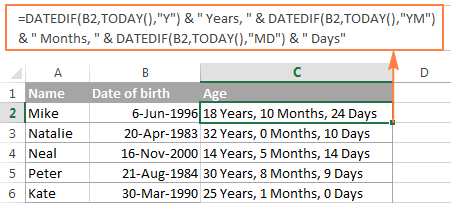To learn other methods of converting a birthdate to age, check out How to calculate age from date of birth.

## Date & Time Wizard - easy way to build date difference formulas in Excel

As demonstrated in the first part of this tutorial, Excel DATEDIF is quite a versatile function suitable for a variety of different uses. However, there is one significant drawback - it is undocumented by Microsoft, meaning, you won't find DATEDIF in the list of functions nor will you see any argument tooltips when you start typing a formula in a cell. To be able to use the DATEDIF function in your worksheets, you have to remember its syntax and enter all the arguments manually, which might be a time-consuming and error-prone way, especially for beginners.

Ultimate Suite for Excel changes this radically as it now provides the Date & Time Wizard that can make almost any date difference formula in no time. Here's how:

1. Select the cell where you want to insert the formula.
2. Go to the Ablebits Tools tab > Date & Time group, and click the Date & Time Wizard button:3. The Date & Time Wizard dialog window shows up, you switch to the Difference tab and supply data for the formula arguments:
• Click in the Date 1 box (or click the Collapse Dialog button to the right of the box) and select a cell containing the first date.
• Click in the Date 2 box and select a cell with the second date.
• Select the desired unit or combination of units from the Difference in drop-down menu. As you do this, the wizard lets you preview the result in the box and the formula in the cell.
• If you are happy with the preview, click the Insert formula button, otherwise try different units.

For example, this is how you can get the number of days between two dates in Excel: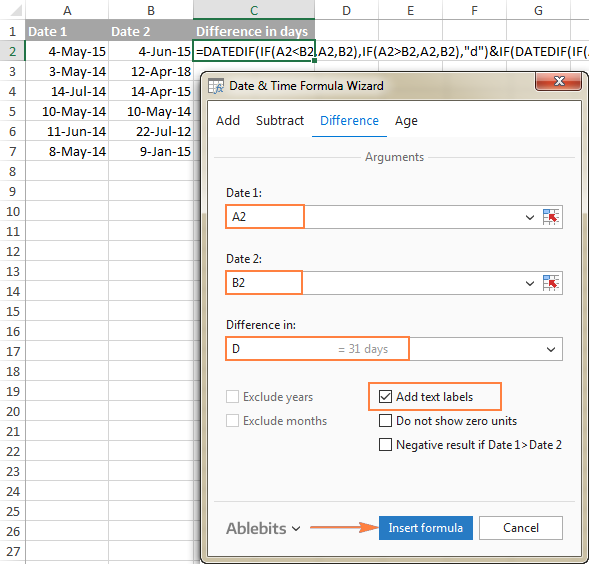Once the formula is inserted in the selected cell, you can copy it to other cells as usual by double-clicking or dragging the fill handle. The result will look similar to this: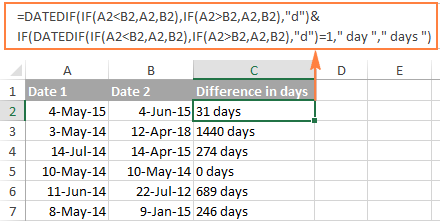To present the results in the most suitable way, a few more additional options are available:

• Exclude years and/or exclude months from calculations.
• Show or do not show text labels like days, months, weeks, and years.
• Show or do not show zero units.
• Return the results as negative values if Date 1 (start date) is greater than Date 2 (end date).

As an example, let's get the difference between two dates in years, months, weeks and days, ignoring zero units: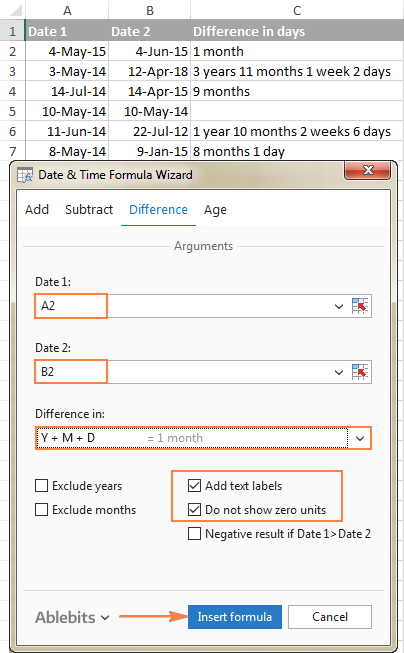### Benefits of using Date & Time Formula Wizard

Apart from speed and simplicity, the Date & Time Wizard provides a few more advantages:

• Unlike a regular DATEDIF formula, an advanced formula created by the wizard does not care which of the two dates is smaller and which is larger. The difference is always calculated perfectly even if Date 1 (start date) is greater than Date 2 (end date).
• The wizard supports all possible units (days, weeks, months and years) and lets you choose from 11 different combinations of these units.
• The formulas the wizard builds for you are normal Excel formulas, so you are free to edit, copy or move them as usual. You can also share your worksheets with other people, and all formulas will remain in place, even if someone does not have the Ultimate Suite in their Excel.

This is how you compute the difference between two dates in various time intervals. Hopefully, the DATEDIF function and other formulas you've learned today will prove useful in your work.

## You may also be interested in

1. Hello!

How do I calculate the number of weeks within a range, that fall within a range?

Scenario:
> I am paying out bonuses based on Number of Weeks Worked to any staff employed during a specific period: Feb 2, 2020 to April 30, 2022 ("Bonus Pay Period")
> I used the DATEDIF formula to get the Total Number Of Weeks Employed for each employee, based on their individual Employment Start Date and Employment Termination Date
> How do I determine how many of those weeks qualify for the Bonus Pay Period?

I've considered calculating the number of weeks each employee worked that falls OUTSIDE the Bonus Pay Period and subtracting that from the Total Number of Weeks Employed number. Is there another way of doing this?

(There are hundreds of employees with very different start and end dates so doing any part of this manually would be very time consuming.)

Thanks for the help!

2. Problem:
If i want to calculate the YY-MM-DD between two dates, i am getting the difference without inclusion of last day.
Example:
Employment Tenure of Mr. XYZ is From 01-12-2020 to 01-06-2023.
I am applying following formula in Excel:
=DATEDIF(D2,E2,"Y") & " Years, " & DATEDIF(D2,E2,"YM") & " Months, " & DATEDIF(D2,E2,"MD") & " Days"
In result i get => 2 Years, 6 Months, 0 Days
My Question is that this function why not calculate the last day that should be 1 Days instead of 0 Days.
Any possible solution for this please share the function.

Regards

• I have explained many times on this blog that a date without a time means the beginning of the day, not the end of the day. For example, 01-06-2023 is 01-06-2023 00:00:00

3. If I have a promotion running from week 8 to week 12, is there a way to count the weeks the promotion is running for but display each of these as opposed to just the total number of weeks? e.g. display week 8, 9, 10, 11 & 12 as opposed to the answer displaying 5 weeks?

• Hi! Count the number of weeks between the two dates as described in the article above. Determine the number of the first week using the WEEKNUM function. Use the SEQUENCE function to create a sequence of numbers starting from the first week.

=SEQUENCE(INT(DATEDIF(A1,A2,"d")/7),,WEEKNUM(A1),1)

4. How to calculate days spend and days left in excel.

5. How do i calculate days from a specific due date including weekends using Network days formula.

6. Please how to input the formula if I want to calculate the number of days a product had been stored in the warehouse from production date till recent day... More so, if that number of days can continue to adjust itself if the item is still in storage.

• Hi! To calculate the number of days, use the DATEDIF function as recommended in the article above. You can get the current date using the TODAY() function. All the information you need is in the article above.

7. Hi. I am doing a vacation planner where staff get extra vacation days after 5 full years service. The vacation year runs from April 01 and only full years are counted. ie if they started in June, the full years entitlement don't start accruing until next April

I just need to find the next time April 1st occurs after the start date, but can't get a reliable result between those who started last year and those who started this year.
eg start date 20/02/2022 =DATE(YEAR(B11)+1,4,1) gives 01/04/2023 when it should be 01/04/2022, but =DATE(YEAR(B11)+0,4,1) will be wrong for those who started last year

8. Hi, How can calculate days differences between two columns if we need to check the year of two columns and if they are in the same then calculate the differences. .
fx. 30-01-2022
30-05-2022

• Hi!
To extract the year from a date, use the YEAR function. Compare the years and calculate the date difference as described in the article above.

9. I am looking for a formula to calculate difference in days between two columns (start and end dates) however some start dates are blank and need to be excluded. Please what formula should I apply?

• Hello!
Use the IF function to conditionally calculate date differences.

=IF(A1="","",DATEDIF(A1, B1, "d"))

10. Hello,

Can you tell me please if possible to calculate average number of days based on criteria such as different product numbers such as average using DATED IF combined (Nestled Argument)? Can DATED IF be used together with Trend and Forecast fucntion too?

Example:

CODE: E35006CE DATE:12/12/12/
CODE: E35006CE DATE:14/12/12
CODE: E35006CE DATE:16/12/12
CODE: E35006CE DATE:17/12/12
CODE: E3513 DATE:1/12/12
CODE: E3513 DATE:5/12/12
CODE: E3513 DATE: 7/12/12

Thank you

11. Date Time Elapsed Time
Dep. Arr.

01.06.2021 3:30 AM
02.06.2021 12:00 PM 0:00

Please give formula for these date clculation
means one person trevel start from date 01.06.2021 & time is 3:30 AM and end date 02.06.2021 & time 12:00 PM
what elapsed time between two date....

• Deduct a2-a1
Format cell as time 37:30:50

12. If I found paperwork on March 9th 2021 what day will I receive it if I have to wait 16 weeks

13. Thanks Svetlana, for your nice teaching.

14. How to create a formula ( using IF) which can select number of days within the particular month from the two column dates which will vary to a year - as below ;

Start * End * no. of days in Jan * no. of days in Feb * no. of days in March* no.of in Aprl
26-Jan-21* 11-Feb-21- ..................... ............... .... ............... ..........
3-Feb-21* 28-Feb-21 ...................... ....................... ............... ...........
5-Feb-21* 25-April-21 ...................... ....................... ............... ..............
20-March-21*2-June-21 ...................... ...................... ................ ...............
25-March-21*28-July-21 ........................ ....................... ................ ...............

15. I want difference between two days should be divided into months & days
e.g.
01-MAR-2020 - 30-Apr-20
basis datedif formula the result is coming as "1" months where as it should come as "2" months

• Hello!
Pay attention to the previous comment just above. There is an answer to your question.

16. In the following example 01/01/2020 - 31/08/2020 - DATEDIF returns 7 months instead of 8. Does anyone have a solution to this problem?

• Hello!
DATEDIF returns the number of full months between dates. In your example, the difference is 7 months and 30 days. The second date is 31/08/2020 00:00:00. That is, this day has not begun.

17. Hello. Can you please help me figure out how to count how many times multiple installations date ranges occur each in week of each year between two date columns for each installer?

I'm trying to count how many, let say "installs," occur per week between the start date and end date columns.
There is more than one row with start dates and end dates that overlap, and I'm trying to break it down by how many are overlapping in each week.
Column B & C are the start and end of the install. E and F are just part of my model.
For example, someone may have 44 installs per year, but how many are occurring each week at the same time.
I'm trying to show how many installs occur/overlap to the right of columns E and F via column G.

See tab SE Grid (DEB). I'm pretty sure the numbers are correct, but you can double-check.
Here are some formulas I have tried:
1. =SUMIFS(\$H\$3:\$H\$44,\$G\$3:\$G\$44, >=K3&)+SUMIFS(\$H\$3:\$H\$44,\$G\$3:\$G\$44, "&K2,\$A\$2:\$A\$217,\$H\$2:\$H\$217,"=K3"},0))*ISNUMBER(MATCH(\$H\$3:\$H\$44, {"=K4),--(\$G\$3:\$G\$44"&WEEKNUM(N3),\$L\$3:\$L\$44,"="&D1,F5:F18,"<="&D2)

18. Hi
Presuming i have 2 dates 1 Apr 2019 in (C3) and 31 Mar 2020 in (D3). I want to calculate the duration of of service in Years, Months and Years. I used the following formula :
=DATEDIF(C3,D3,"y")& " Years, "&DATEDIF(C3,D3,"YM")&" Months and " &DATEDIF(C3,D3,"md")& " days"
The answer i got was (2 years 11 months and 30 days) I want the date to show as (3 years, 0 months and 0 Days)
How do i go around this?

Thank you

Tessie

• Hello Tessie!
I am not sure if this is logical, but you can use the formula

=IF(DATEDIF(C3,D3,"md") < 30, DATEDIF(C3,D3,"Y")& " Years, "&DATEDIF(C3,D3,"YM")&" Months and " &DATEDIF(C3,D3,"md")& " days",DATEDIF(C3,D3+1,"Y")& " Years, "&DATEDIF(C3,D3+1,"YM")&" Months and " &DATEDIF(C3,D3+1,"md")& " days")

I hope this will help, otherwise please do not hesitate to contact me anytime.

• I have an csv data I have to calculate the days between the order date and the ship date.
i have used some formulas but seem not to be working for me.
thank you

• Hello!
When importing data from CSV, the date is recorded as text. I can't see your data, so I recommend using the instructions above.

19. Hy if i have two dates i.e
01/04/2020 to 10/08/2020 then how to bifurcate difference between two dates into different months i.e in April month-30 days, May month 31days, June 30 days , July 31 days, Aug 10 days

• Hello Dishant!
I hope you have studied the recommendations in the above tutorial. Please let me know in more detail what you were trying to find, what formula you used and what problem or error occurred. In that case I will try to help you.

20. I used the DATEDIF function to calculate the difference between 2 dates but when the value is negative it displays error. How can I correct it?

• Hello Aminu!

=IFERROR(DATEDIF(A1,A2,"d"), DATEDIF(A2,A1,"d"))

Hope you’ll find this information helpful.

• Same issue, but cannot seem to make the formula work. My original formula is =DATEDIF(C3,D3, "d") & " days"
And it works if C3 is lets say 01May2023 and D3 is 04 May 2023.
But if the date in C3 is 04May2023 and D3 is 01May2023 it should display -3 days but I get an error.

• The DATEDIF function cannot return a negative value. Read Example 1 above carefully.

• Hi, please help as I found negative value as the result of the DATEDIF function as follows :

Presuming I have 2 dates 31 May 2014 in (A1) and 1 Mar 2015 in (B1), I use the function : =DATEDIF(A1,B1,"md") and why it returns -2 as the result ?

Then I try to use longer function :
=DATEDIF(A1, B1, "y") &" years, "&DATEDIF(A1, B1, "ym") &" months, " &DATEDIF(A1, B1, "md") &" days"
And why it returns :

0 years, 9 months, -2 days ?

Is the "-2" days value considered an excel calculation error as there should be no negative value for date difference ?

21. hello
i create excel in a sheet
what i need is to some all items with the same date alone example whole 01/january alone and 02 january alone and finally add up between 01/january and 02/january together in the month of january

22. Hi,
I have 24 columns with Jan' 20 to Dec' 21 and having some values in rows now I wish to transfer values to another sheet in columns (Jan'20 to Dec'21) by adding days different for all rows like 1, 10, 15, 30, 45, 60, 75, 90 etc.
For Eg. If I have 20 in Jan'20 and need to add 45 days in Jan' 20 then another sheet should have 20 in Feb' 20 or I have to add 90 days then 20 should go to Mar' 20 or Apr' 20.

23. Hi guys,
how to i calculate between two years

example: 2years 4months and 5years 3 months

24. Please send the the formula for following conditions
If the date is between 2 Jan 2019 and 1 July 2019 the result would be 1 Jan 2019

25. Hi, I need a help to find Time Different of Two Dates with time but its should be with in two other Date and Times. Eg. END Time : 12/18/2019 23:16 - Start Time : 12/18/2019 21:16 but it would in bracket of available Time 12/18/2019 22:16
and 12/18/2019 22:26 . Ans: 0:10:00 ; How can I reach ?

26. In datedif formula the days should not come as "30"
eg.486 days = 1 year 3 month 30 days (in datedif formaula)
but we want the answer is 1 year 4 month .

27. if i have a date range 10/01/2019 - 11/19/2019, what formula will calculate the number of days in the first month only? It would also need to consider if the date range is 10/01/2019 - 10/05/2019.
Many thanks,
B

28. Someone please can guide me how i can extract required text from below data as i need Model name i.e. Smsng, ID Iphone x, Code125, EMEI, EMI, ID.

Code125 EMEI2546 Alb Dist. Iphone x user Alph.
ID36520 2564MEI Smp. Lng Smsng met 2456
ID36520 2564MEI Colt. Conc. local mkv 365
ID36520 EMEI2546 Alb Dist. Smsng mkl 25
Code125 2564MEI Smp. Conc. Iphone x user 14
Code125 2564MEI Smp. Lng local met 896
ID36520 2564MEI Alb Conc. Iphone x mkv 125
Code125 2564MEI Smp. Dist. Smsng mkl 35
ID36520 EMEI2546 Alb Lng local user 46
ID36520 2564MEI Smp. Dist. Smsng met 125
Code125 EMEI2546 Colt. Mnt. Iphone x mkv 400
Code125 2564MEI Alb Lng local user 600
Code125 EMEI2546 Alb Dist. Iphone x met 1915
ID36520 2564MEI Con Lng Smsng user 16

29. If i have start date & end date available in column so mentioned formula is use full to calculate the days, but if any column only start date mention and end date is not available & i want to know how many days have passed from start date. any formula where we can use both formula's to calculate the same.
Start Date & date : =DATEDIF(H2,I2,"D")
only Start date : =TODAY()-H2

How we can use both formulas

• Hello,
Did you recieve a response to this query as I am looking for a formula to do the exact same?

30. Dear,
I have same question like Rab Nawaz.
I have to count different data, like as shown on the right side here (only count Years, Months and days)
01 September 1994 31 October 1994 0Years, 1Months, 30days
04 September 1995 30 November 1995 0Years, 2Months, 26days
01 December 1995 30 May 1996 0Years, 5Months, 29days

Thank You beforehand.

31. Dear,
I have same question like Rab nawaz.
I have count different data like as
01 September 1994 31 October 1994 0Years, 1Months, 30days
04 September 1995 30 November 1995 0Years, 2Months, 26days
01 December 1995 30 May 1996 0Years, 5Months, 29days

32. 01/07/2019 20:30 to 08/07/2019 09:55
how to calculate time

33. How to write at a time if conditions and eomonth in excel. I am write a one condition =if(C1=“FALSE”,EOMONTH(D3),B40,””) but this is wrong plz tel me correct answer (C1=Cell address, D3=Cell address, B40=Cell address there another one date)

34. Hi guys,
Can you help me with something ?
i want to compare 2 calendar dates in excel , for example:
DATE 1 - DATE 2
10/3/2017--2/6/2020
1/17/2019--1/17/2020
9/25/2017--1/25/2019
3/21/2018--3/21/2019
as some of the days have been done in the past I would need to compare the dates between themselves and between "today"'s date to have am update of the process any time ;as this dates represent completion days of our colleagues training.
DATE 1 is the date when the training has been completed
DATE 2 is the due date for the next training
If the date is DATE 2 < TODAY by 3 moths range it will be highlighted with red
if the date is DATE 2 TODAY it will be highlighted with amber/yellow
if the date is DATE 2 > TODAY it will be highlighted in green
can you guys help me :)

35. Calculate number of date between dates and days in Excel

36. Hi,
I have confusion :- my start date is - March 01, 2019 and end date - today (March 27, 2019) if i set formula it is calculating 26 Days but if i count it manually it is calculation 27 Days. I don't understand what is my problem.... Please provide me a solution......

37. I'm trying to track evaluations. I want the same month and day as their date of hire, but this year to be included. For example, date of hire: 2/14/1997, next eval: 2/14/2019. Is there a formula for this?

38. hi
i am not able to use this formula in windows10

=DATEDIF(M89, N89, "y") &" years, "&DATEDIF(M89, N89, "ym") &" months, " &DATEDIF(M89, N89, "md") &" days" it showing #value!
and same for this also
=(MID(T88,4,2)&"/"&LEFT(T88,2)&"/"&RIGHT(T88,2))+0

kindly help on it

39. I have a date i.e 22-Mar-1968 and I want to add in this date only 23 year. what will be the formula in excel. please guide me.

• Please reply my question posted on 12 February 2019. With Thanx

40. I need to calculate a % of a task completed within 5 days. In brief:
* Column J = Notified Date
* Column M = Completed Date
* Column N = work days it took to complete task
KPI is 5 Days, so I need to work out from Column N if this was within 5 days, ie. what the % rate was. If 5 days or less, then response would be 100%. If less than 5 days, then display the % rate.
Is there a formula that can calculate this %. Thanks :)

41. How to calculate number of exists days for the below dates
09/04/2018 12:50 20/04/2017 11:55

Please solve my problem as soon as your free time Thanks... :)